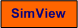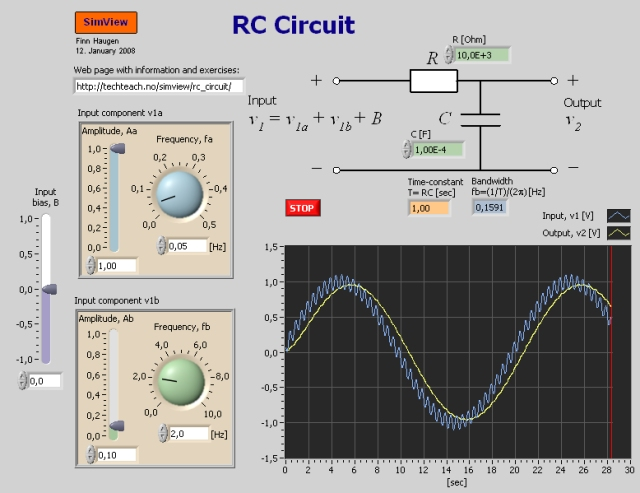# RC-Circuit

Snapshot of the front panel of the simulator:• What is needed to run the the simulator? Read to get most recent information!
• Tips for using the simulator.
• The simulator: . The simulator runs immediately after the download by clicking Open in the download window. Alternatively, you can first save a copy of the exe-file on any directory (folder) on your PC and then run the exe-file, which starts the simulator.

## Description of the simulated system

In this simulator an RC-circuit is simulated. It consists of a resistor R [Ohm] and a capacitor C [Farad] connected in a circuit, see the front panel of the simulator.

In the tasks below the dynamic properties of the RC-circuit will be observed through simulations. In the simulator the input signal is a sum of two independent sinusoids and a bias (a constant).

## Aim

The aim of this simulator is to increase the understanding of the RC-circuit as a dynamic system.

## Motivation

In applikations where a simple analog lowpass filter is needed, the RC-circuit is commonly used, as in I/O equipment (input/output) for attenuation of measurement noise.

## Mathematical model

It can be shown that the relation between the input voltage v1 and the output voltage v2 is given by the following differential equation:

(1) RC*dv2/dt = v1 - v2

By taking the Laplace transform of this differencial equation we find the following transfer function, H(s), from v1 to v2:

(2) H(s) = 1/(Ts+1)

where

(3) T = RC [s]

is the filter time constant.

Using frequency response theory, it can be found that the bandwidth of the filter is

(4) fb = (1/T)/(2p) [Hz]

Unless otherwise stated you should use default values of the various parameters (you get the the default value via right-click on the front panel element).

1. The step resonse of the filter: In this subtask, you should suppress the sinusoids (by setting the amplitudes to zero).
1. Calculate (by hand) the time constant T according to Eq. (3) above. Is the result the same as can be seen on the front panel of the simulator when the simulator runs? Then run a simulation where you adjust the signal component B as a step, and read off the time constant from the response. Is the observed time constant the same as the calculated time constant?
2. Run a simulation with some constant input signal, say V1. What is the corresponding steady-state value, v2s, of the output voltage response? From these results, what is the relation between V1 and v2s? Can you calculate this relation directly from the model (1)?

2. Frequency response of the filter: Set the signal component B to zero. Use default values of R and C.
1. Let the sinusoid v1a have amplitude 0.5 and frequency 0.05Hz, and let sinusoid v1b have amplitude 0.5 and frequency 1Hz. Thus, signal component v1a has a smaller frequency than the bandwidth, which is 0.16Hz, while the component v1b has larger frequency than the bandwidth. In other words, v1a are in the passband of the filter, while v1b is in the stoppband of the filter. Run the simulator! Can you observe from the simulation that signal component  that component 1 is in the passband, while v1b is in the stoppband?
2. The bandwidth is defined as the frequency where the amplitude gain of the filter is 1/sqrt(2) =  0.71 = -3dB. In other words, if the sinusoidal input signal has frequency equal to the bandwidth, the amplitude of the output signal is 71% of the amplitude of the input signal. Verify this by running a simulation.

[SimView] [TechTeach]

Updated 27 August 2017. Developed by Finn Haugen. E-mail: finn@techteach.no.